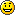# Fibonacci Fractions

I think that it is really cool that some fractions have interesting patterns in their decimal expansion.  For example,

$$1/499 = 0.0020040080160320641282565130260….$$

You can see the first 8 values of the doubling sequence (a.k.a the powers of 2)  2, 4, 8, 16, 32, 64, 128, and 256 in the decimal expansion.  If you want more values, you can just add more 9’s to the denominator.

\begin{aligned} 1/49999 = &0.0000200004000080001600032000640012800256005120\\&1024020480409608192163\ 8432768655373107462….\end{aligned}

Other fractions can encode other sequences.  The first several square numbers 1, 4, 9, 16, … are encoded by the fractions

$$\small\frac{10100}{970299},\frac{1001000}{997002999},\frac{100010000}{999700029999},\frac{10000100000}{999970000299999},\frac{1000001000000}{999997000002999999},….$$

For example,

$$\frac{1001000}{997002999} = 0.001004009016025036049064081100121144169196225….$$

It turns out that it is not too hard to find sequences of fractions which encode any sequence of positive integers that is a sum of

• polynomials  (e.g.  cubes         1, 8, 27, 64, 125, …),
• exponentials (e.g. powers of 3  1, 3, 9, 27, 81, 243, …), or
• products of polynomials and exponentials.  (e.g.  cubes times power of 3     1, 24, 243, 1728, 10125, 52488, 250047,…).

### The Fibonacci sequence

The Fibonacci sequence is

1, 1, 2, 3, 5, 8, 13, 21, 34, 55, 89, 144, 233, 377, 610, 987, 1597, 2584, 4181, 6765, ….

Each value after the initial ones is the sum of the previous two values (e.g. 2 +3 =5, 3+5 =8, …).  It turns out that the Fibonacci sequence is the sum of exponentials, so it also can be encoded in a sequence of fractions.

\begin{aligned} \frac{1000}{998999} &= 0.00100100200300500801302103405509… \\ \frac{10000}{99989999} &= 0.000100010002000300050008001300210… \\ \frac{100000}{9999899999} &= 0.0000100001000020000300005000080001… \\ \frac{1000000}{999998999999} &= 1.000001000002000003000005000008000013…\cdot 10^{-6} \\ \frac{10000000}{99999989999999} &= 1.000000100000020000003000000500000080000013\cdot 10^{-7} \\ \end{aligned}.

All of these fractions $f_k$ can be computed with the formula

$$f_k=\sum_{i=1}^\infty \frac{a_i}{10^{\ k i}}$$

where the $a_i$ are the values of the sequence and $k$ is a positive integer specifying the spacing between the values of $a$ in the decimal expansion.   This sum is fairly easy to compute when $a$ is a polynomial, an exponential, or a combination of polynomials and exponentials.

For example, to get the doubling numbers $a_i = 2^i$, the fractions would be

\begin{aligned}\sum_{i=1}^\infty \frac{a_i}{10^{\ k i}}&= \sum_{i=1}^\infty \frac{2^i}{10^{\ k i}}\\&= \sum_{i=1}^\infty \left(\frac{2}{10^k}\right)^i\\&=\frac{\frac{2}{10^k}}{1-\frac{2}{10^k}}\quad{\text{using} \sum_{i=1}^\infty x^i= \frac{x}{1-x}}\\&= \frac{2}{10^k – 2}.\end{aligned}

For the Fibonacci sequence $$a_i = \frac{\varphi^i-\psi^i}{\varphi-\psi}= \frac{\varphi^n-\psi^n}{\sqrt 5}$$ where $$\varphi = \frac{1 + \sqrt{5}}{2} \approx 1.61803$$ and $$\psi = \frac{1 – \sqrt{5}}{2} = 1 – \varphi = – {1 \over \varphi} \approx -0.61803.$$ (Copied verbatim with glee from the Wikipedia).

The math required to compute the Fibonacci fractions is almost the same as the powers of two math using the same $$\sum_{i=1}^\infty x^i= \frac{x}{1-x}$$ trick.After you go through the computations, the Fibonacci fractions can be found with the formula

$$f_k = \frac{10^k}{10^{2 k} – 10^k – 1}.$$